# Immersion

(diff) ← Older revision | Latest revision (diff) | Newer revision → (diff)
A mappingof one topological space into another for which each point ofhas a neighbourhoodwhich is homeomorphically mapped ontoby. This concept is applied mainly to mappings of manifolds, where one often additionally requires a local flatness condition (as for a locally flat imbedding). The latter condition is automatically fulfilled if the manifoldsandare differentiable and if the Jacobi matrix of the mappinghas maximum rank, equal to the dimension ofat each point. The classification of immersions of one manifold into another up to a regular homotopy can be reduced to a pure homotopic problem. A homotopy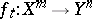is called regular if for each pointit can be continued to an isotopy (in topology)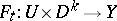, whereis a neighbourhood of,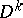is a disc of dimension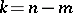and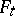coincides with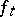on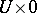, where 0 is the centre of the disc. In the differentiable case, it is sufficient to require that the Jacobi matrix has maximum rank for eachand depends continuously on. The differential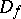of an immersion determines a fibre-wise monomorphism of the tangent bundle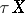into the tangent bundle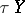. A regular homotopy determines a homotopy of such monomorphisms. This establishes a bijection between the classes of regular homotopies and the homotopy classes of monomorphisms of bundles.
The problem of immersions in a Euclidean space reduces to the homotopy classification of mappings into a Stiefel manifold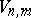. For example, because, there is only one immersion class of the sphere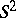into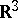, so the standard imbedding is regularly homotopic to its mirror reflection (the sphere may be regularly turned inside out. Because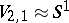, there is a countable number of immersion classes of a circle into the plane, and because the Stiefel fibration overis homeomorphic to the projective spaceand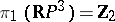, there are only two immersion classes from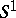into, etc.
For figures illustrating the fact thatcan be regularly turned inside out see [a3].